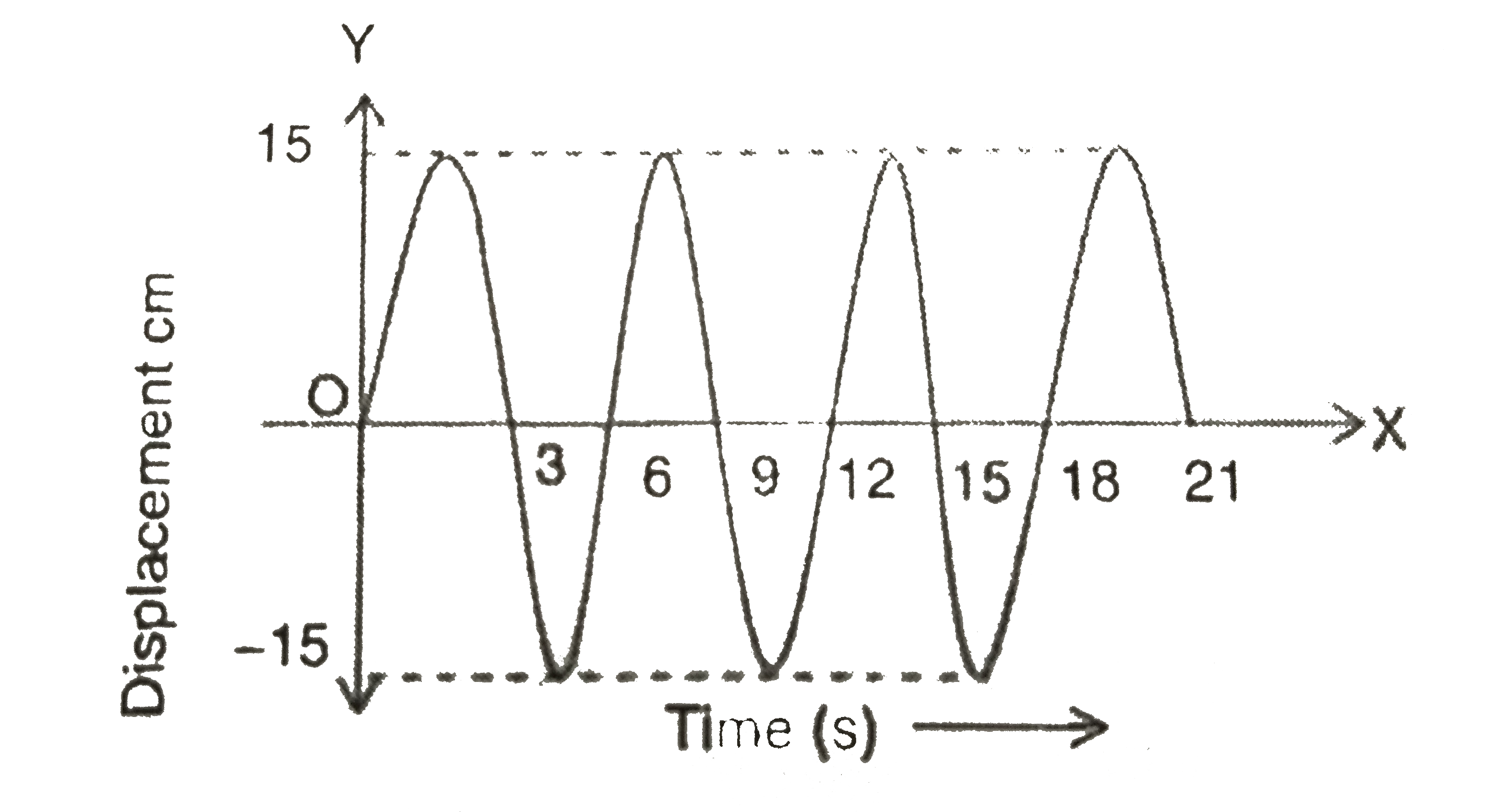# In the case of a simple pendulum, a graph is drawn between the displacement and time taken for its oscillations as shown in the figure . Then From the

10 views
in Physics
In the case of a simple pendulum, a graph is drawn between the displacement and time taken for its oscillations as shown in the figure . Then
From the given figure, the number of oscillation performed by the bob at the end of 21 s is ________A. 3
B. 4
C. 2
D. 3^(1//2)

by (25.0k points)